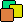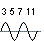Prime I.T. Help Prime I.T. :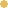Bitcoin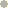Litecoin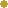DodgecoinVersion Française

# The Sieve of Eratosthenes

Note : This article has been translated using an automatic translator and one pass was made to remove biggest errors.
If you want to contribute to the readability of this article, you can notify me translations errors (a word, a sentence, a paragraph) by contacting me at the email address at the bottom of the page. Thanks

## The principle

The Sieve of Eratosthenes is a method for finding all prime numbers up to a fixed number N.
To do this, we must take the numbers from 2 to N and successively eliminate all those multiples of an integer and so not prime (non-prime number is called composite).

The elimination works in the following way:

• Take the first element of the array, ie 2 and eliminate all multiples of 2 of the table.
• Then take the next number not eliminated, here 3 and remove multiples of 3.
• Then take the next number not eliminated, here 5 (because 4 was eliminated in the first step) and eliminate multiples of 5.
• and so on...

At the end all non-primes are eliminated

## First implementation

From an algorithmic point of view, we need several things :

• An array of Boolean 2 to N as to whether a number is removed or not.
• A loop over this table.
• A second nested loop eliminating multiple for a selected number.

This gives the first version relatively naive (written in java) :

private static final int MAX = 100000000;
private static boolean[] array = new boolean[MAX];
//--//
for (int i = 2; i < MAX; i++) {
if (!array[i]) {
int j = i + i;
while (j < MAX) {
array[jtrue;
j += i;
}
}
}

Note : Throughout the presentation of the algorithms, we keep the same formalism for "key" variables, namely :

• array represents the array of booleans with true meaning eliminated (it is a composite number) and false not eliminated (this is potentially a prime).
• MAX is the maximum size of the list of primes that we want to find (the limit set N).

On an old machine 2Ghz AMD 3200+ and java (not necessarily known for its velocity), we arrive at a speed already interesting. Pi(x) represents the number of primes up to x.

MAXPi(MAX)Time (sec)
1 000 00078 4980.031
10 000 000664 5790.657
100 000 0005 761 4557.578

Which means, we found 5.7 million prime numbers between 2 and 100 milion in 7 seconds. source
It's good but not enough...

## Second implementation

This first version of the algorithm is widely optimizable. Indeed it is possible to work on two areas of improvement namely, find the numbers faster by accelerating the processing speed (double loop should be faster) and/or expand the range to find more prime number (enlarge the limit N)

For processing speed, it "simply" that the 2 loops do not test the numbers that are already trivially eliminated... Ok but how ?
If we unfold The algorithm by hand, we have :

• Take the number 2 and eliminate multiple : 2*2, 2*3, 2*4, 2*5, 2*6...
• Take the number 3 and eliminate multiple : 3*2, 3*3, 3*4, 3*5, 3*6...
• Take the number 5 and eliminate multiple : 5*2, 5*3, 5*4, 5*5, 5*6...
• Take the number n and eliminate multiple : n*2, n*3, n*4, n*5, n*6...

We see that the algorithm tries to remove too many multiples, for example 3*2 of step 2 has been removed in step 1 (2*3), and 5*2, 5*3 of step 3 with 2*5 of step 1 and 3*5 of step 2. In conclusion, for a given number n, it is useless to try to eliminate multiple below its square (n*n) because they have already been eliminated in previous rounds. This will result in an increase of the lower bound of the second loop that goes "i+i" to "i*i" and a lowering of the upper limit of the first loop that passes to square root of N.

For the second point optimization of the expansion of the limit N, we will not save even numbers. Indeed, except for the number 2 all other even numbers are composite. With this obvious trick we divide the storage by two.

This gives the second version :

private static final int MAX = 100000000;
private static final int SQRT_MAX = (intMath.sqrt(MAX1;
private static final int MEMORY_SIZE = (int) (MAX / 2);
private static boolean[] array = new boolean[MEMORY_SIZE];
//--//
for (int i = 3; i < SQRT_MAX; i += 2) {
if (!array[(i / 2)]) {
int j = (i * i2;
while (j < MEMORY_SIZE) {
array[jtrue;
j += i;
}
}
}

Overall we divide the time by 2.

MAXPi(MAX)Time (sec)
1 000 00078 4970.016
10 000 000664 5780.281
100 000 0005 761 4543.063

Most assiduous note that we lose one prime number compared to the first implementation (column Pi). Just because this algorithm does not store the even numbers and so 2 is omitted. source

## Third implementation

With this second implementation, we see that we reach a fundamental limit of the Sieve of Eratosthenes. Without touching java jvm parameters, if we try to reach 1 billion, we get :

java.lang.OutOfMemoryError: Java heap space
at org.cpas.prime.Eratos2.<clinit>(Eratos2.java:12)

The algorithm needs a lot of memory to run because we need to store N booleans (or N/2 in version 2). The memory complexity of the Sieve of Eratosthenes is O(n).
Therefore try to store more intelligently. Instead of having an array of booleans we can pass to a binary representation where each bit represents a number removed or not removed. The support binary will be a byte java equal to 8 bits.

private static final long MAX = 1000000000L;
private static final long SQRT_MAX = (longMath.sqrt(MAX1;
private static final int MEMORY_SIZE = (int) (MAX >> 4);
private static byte[] array = new byte[MEMORY_SIZE];
//--//
for (long i = 3; i < SQRT_MAX; i += 2) {
if (!getBit(i)) {
long j = (i * i);
while (j < MAX) {
setBit(j);
j += (* i);
}
}
}
//--//
public static boolean getBit(long i) {
byte block = array[(int) (i >> 4)];
byte mask = (byte) (<< ((i >> 17));
}

public static void setBit(long i) {
int index = (int) (i >> 4);
byte block = array[index];
byte mask = (byte) (<< ((i >> 17));
}

And we reach a billion....

MAXPi(MAX)Time (sec)
1 000 00078 4970.031
10 000 000664 5780.203
100 000 0005 761 4543.047
1 000 000 00050 847 53335.625

## Fourth implémentation

This is all well and good but this limit space is always present. As clever as we can be, we still have to allocate an array of N elements for N large enough exceed the memory size of the computer.
Do not go buying memory in computer shop, the solution is segmented sieve of Eratosthenes.

The idea is to split the table into X pieces (segments) and run the algorithm of the sieve on each of these pieces. Not only it is possible but it does not more "leaden" too performance of the application (the processing time of each segment is roughly equivalent to the computation time of the third implementations).
Following optimization of the second implementation, we know that to find all prime numbers from 2 to N, we can simply apply the sieve from 2 to square root of N. This is not trivial because for finding all primes between 2 and 10 billion, we need only go up to 100 miles. The purpose of segmented Eratosthenes is to compute the list of prime numbers up to the square root of N (initiation) and "sieve different segments" with this initiation.
By cons optimization on the course numbers in the second loop changes. In the case of a segmented Eratosthenes is more difficult to implement because the beginning of the "sieve" starts at the beginning of the segment that is a number chosen arbitrarily (and not the fixed value "3" like in version 2 or 3). So we pass a "i*i" to "i+i" but it should be possible to do better. Too many neurons are involved to optimize this part, I leave the reader to find the solution...

Interesting note : the segments are independent, it is not mandatory to first calculate the first segment to derive the second. This means that it is possible to calculate the prime numbers between any two thresholds (as long as the initiation is large enough to reach the square root of the upper bound of the segment), it also means that it is quite possible to create a distributed Eratosthenes across multiple computers (each seeking its segment).

Arrays.fill(segment, (byte0);

long debut = numSegment * MAX_SEGMENT;
long fin = debut + MAX_SEGMENT;
long finSqrt = (longMath.sqrt(fin1;

int p = -1;
int i = 1;
while (p < finSqrt) {
p = amorce[i++];
long j = p - (debut % p);
j += ((j & 1== ? p : 0);

long p2 = p * 2;
while (j < MAX_SEGMENT) {
segment[(int) (j >> 4)] |= (<< ((j >> 17));
j += p2;
}
}

numSegment represents the segment number and MAX_SEGMENT the segment size, we conclude with the beginning and end of the segment.

MAXPi(MAX)Time
10 000 000 000455 052 5115mn 24s
100 000 000 0004 118 054 8131h06mn

Finally it should be noted that the calculation of primes for the initiation in Eratos4 is made with trial division. It would have been quicker to do with Eratosthenes (a handful of milliseconds against 500ms) but you have to vary the pleasures ... source

This implementation generates a dump segments and writes files in the form prime_{N° segment}_{start segment}.bin
Here is an example of how to read these files : source

## Fourth implementation (bis)

By analyzing the code Sieve of Eratosthenes segmented we see that it is expensive in computation time at the beginning of the sieve of a segment, when we apply the elimination with small numbers from the initiation. This is because... precisely the numbers are too small and need a lot of "i+i" to reach the end of the segment.
See :

• On a segment of one billion, we must 333 million loop turn to eliminate all multiples of 3.
• For 5, it takes 200 million loop turn.
• For 17, we have 58 million.

We'll do a little optimization by computing one time all the "i+i" for small numbers of initiation (called the mask) then we will apply unchanged during the sieve of a segment. Note that for "apply as is" it will require that the segment size is divisible by all these small numbers. For Eratos4bis we precompute a mask up to 17 and we will locate the segment size to 1000089090 = 3 * 5 * 7 * 11 * 13 * 17 * 3918.
With this optimization algorithm loses 4 seconds at startup (time to calculate the mask) then win back this time for each calculated segment. At the end the performance gain is of the order of 15%.

MAXPi(MAX)Time
10 000 890 900455 091 1364mn 35s
100 008 909 000-To verify-54mn

## Fourth implementation (ter)

Since Java is a bit slow, try to transcribe Eratos4bis in C.

MAXPi(MAX)Time
10 000 890 900455 091 1363mn 35s
100 008 909 000-To verify-35mn

Actually it is much faster !
Tested under Windows 32-bit machine with a simple core, apparently this gain is not so important on modern machines. Maybe java can use the fully machine resources unlike C and so improve performance ? source

## And now ?

It's nice to have calculated billion prime numbers but now what do we do ? One segment of 1 billion represents about a file of 60MB (26MB zipped in). So in less than an hour of computing time, we find all the prime numbers from 1 to 100 billion (there are 4 billion), but above we have 6GB of data (compressed or 2GB).
This algorithm shows that it is relatively fast to generate linearly all primes between 2 thresholds, for against the store and/or use them is another challenge...

A website created by Les composants associés (cpas)